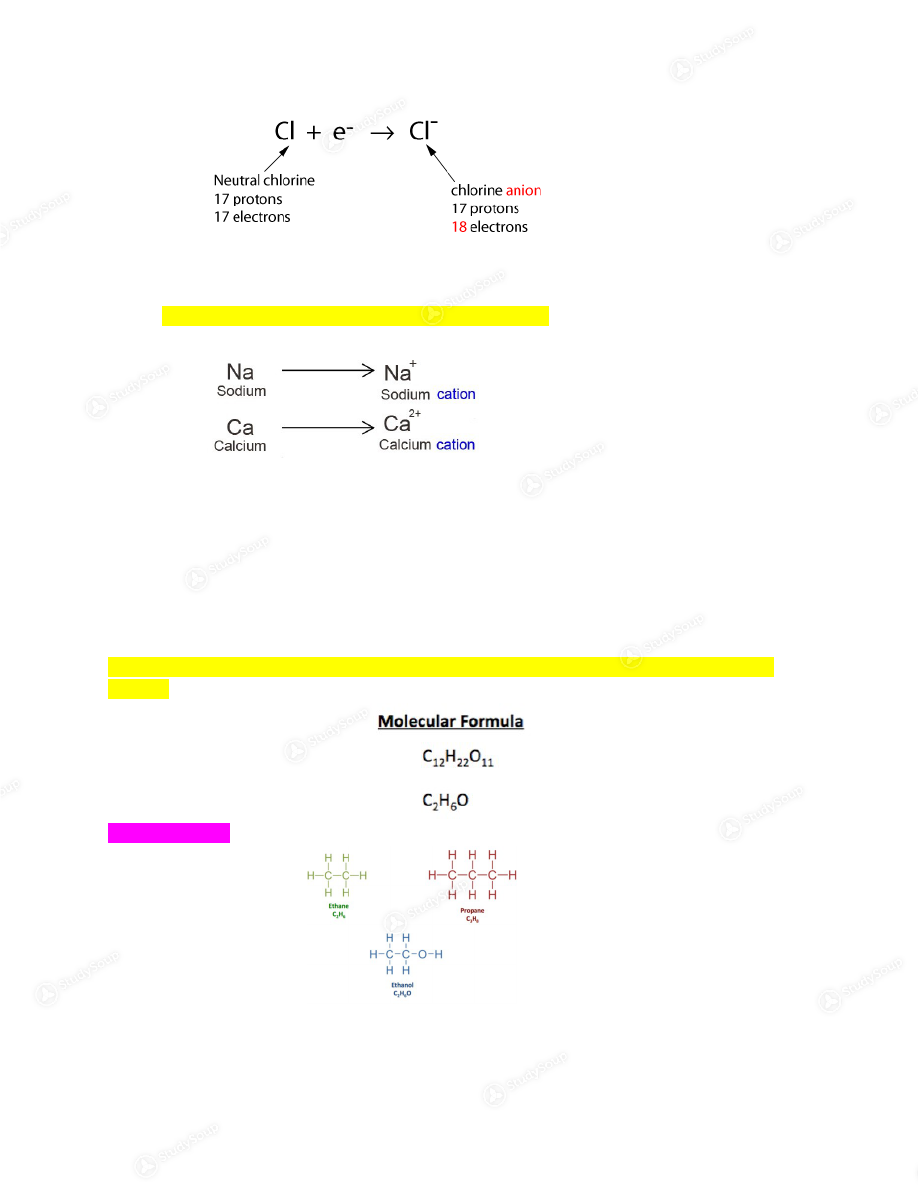Limited time offer 20% OFF StudySoup Subscription details

# CSU - CHEM 111 - Chem 111 Week 3 notes - Class Notes

### Created by: Kristine Emken Elite Notetaker

> > > > CSU - CHEM 111 - Chem 111 Week 3 notes - Class Notes

CSU - CHEM 111 - Chem 111 Week 3 notes - Class Notes

##### Description: - Isotope - Finding atomic mass - Ions - Chemical formula, structural formula, condensed structural formula, ball and stick model, space filling model - Empirical formula - Standard states of elements - Mole - Atomic mass - Masses of molecules and compounds - Kinetic vs potential energy - Potential energies of objects - Potential energies of ions - Definition of amplitude, wavelength, f
0 5 3 75 Reviews
This preview shows pages 1 - 3 of a 12 page document. to view the rest of the content2.3 Continued Isotope­ atoms with same number of protons but a different number of neutrons They are the same element but will have a different mass Isotopic abundance­ percent abundance of each isotype of an element Masses of atoms Atomic mass = the average mass of all isotopes of an element Elemental atomic mass ¿ (Fractional isotopic abundance) × (Isotopic molar mass) Example problem What is the average weight of chromium Isotope Abundance Mass ²Cr 83.79% 51.94 amu ³Cr 9.50% 52.94 amu Cr ⁵⁴ 2.36% 53.94 amu ºCr 4.35% 49.94 amu1. First, we will need to convert our percentages into fraction and then put them into the  equation. Atomic mass is the sum off all isotopes. We find this by multiplying the
abundance (in a fraction) by their mass for each isotope and then add them all together
( 0.8379× 51.94 amu ) + ( 0.0950 × 52.94 amu ) + ( 0.0236 × 53.95 amu ) +( 0.0435× 49.95 amu) 2. *Remember to separate steps* We will do multiplication then addition to keep track of  the number of significant figures 43.520526+5.0293+1.272984+2.172825 3. With the rule for multiplication/division we need to THINK (not round because we still  have another step) about significant figure for all the numbers. For 0.8379 × 51.94 amu  the lowest number of significant figures is 4 meaning the 2 in  43.52 would be the last digit. For  0.0950 × 52.94 amu  the lowest number of  significant figures is 3 meaning the 2 in 5.02 would be the last digit. For 0.0236 × 53.95 amu  the lowest number of significant digits is 3 meaning the 7 in  1.27 would be the last digit. For  .0435× 49.95 amu  the lowest number of significant  figures is 3 meaning the 7 in 2.17 is the last digit. 43.52∨0526+5.02∨93+1.27∨2984 +2.17∨2825 4. Now we will add them together 51.995635 5. We now need to consider the rule for addition/subtraction. Based on where the digit with  uncertainty lies from looking at the rule for multiplication for all four numbers that is in
the hundredths place which is where our answers last digit (showing uncertainty) will be
52.00 6. We can then look at the periodic table of elements to check our answer. *If your answer  is a little off (51.99 or 51.89) that is ok* The periodic table of elements says the atomic mass of Cr is 52.00 Ions­ charged atoms Vary in the number of electrons they have (for the same element) Anions­ negative charged ions that have gained an electron(s)Cations­ positive charged ions that have lost an electron(s) 2.4 Chemical formulas Chemical formula/molecular formula­ describes the exact number of each atom of each element in a
molecule
Structural formula Condensed structural formula

This is the end of the preview. Please to view the rest of the contentJoin more than 18,000+ college students at Colorado State University who use StudySoup to get ahead
12 Pages 30 Views 24 Unlocks
• Notes, Study Guides, Flashcards + More!Join more than 18,000+ college students at Colorado State University who use StudySoup to get ahead
##### Description: - Isotope - Finding atomic mass - Ions - Chemical formula, structural formula, condensed structural formula, ball and stick model, space filling model - Empirical formula - Standard states of elements - Mole - Atomic mass - Masses of molecules and compounds - Kinetic vs potential energy - Potential energies of objects - Potential energies of ions - Definition of amplitude, wavelength, f
12 Pages 30 Views 24 Unlocks
• Notes, Study Guides, Flashcards + More!
Get Full Access to CSU - Chem 111 - Class Notes - Week 3
×
Get Full Access to CSU - Chem 111 - Class Notes - Week 3

I don't want to reset my password

Need help? Contact support

Need an Account? Is not associated with an account
We're here to help